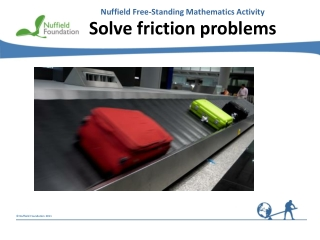DownloadDownload PresentationSolve friction problems

# Solve friction problems

Download Presentation## Solve friction problems

- - - - - - - - - - - - - - - - - - - - - - - - - - - E N D - - - - - - - - - - - - - - - - - - - - - - - - - - -
##### Presentation Transcript

1. Nuffield Free-Standing Mathematics Activity Solve friction problems

2. What forces are acting on the sledge? What force is making the suitcases accelerate?

3. The friction model Before sliding occurs … Frictionis just sufficient to maintain equilibrium and prevent motion F< FMAX On the point of sliding and when sliding occurs … F = mR where F is the friction, R is the normal contact force and m is a constant called the coefficient of friction

4. 5 kg R 15N F 5g 15N Friction problems Example If m = 0.4, will the box move? Think about What is the smallest force that will make the box slide along the table? Solution Vertical forces: R= 5g Maximum possible friction FMAX = m R = 19.6 N = 0.4  5g The pushing force is less than 19.6 N where g = 9.8 ms–2 The box will not move

5. smooth R T F 0.4g 0.2g 1 = 2 0.4g Friction problems Example If the package is on the point of moving, find m. 400 grams Think about What forces are acting on the package? Vertical forces: R= 0.4g 200 grams Solution On the point of moving F= m R = m 0.4g F= T As the pulley is smooth T= 0.2g m 0.4g= 0.2g m = where g = 9.8 m s–2

6. (u + v)t s = ut + at2 s = 2 1 2 More difficult friction problems may require the use of … Think about Why does the friction model allow the use of these equations? Newton’s Second Law Resultant force = mass  acceleration where the force is in newtons, mass in kg, and acceleration in m s–2 Equations of motion in a straight line with constant acceleration v = u + at v2 = u2 + 2as where u is the initial velocity, v is the final velocity, a is the acceleration, t is the time and sis the displacement

7. 20 m s–1 R F 1200g More difficult friction problems Example The car brakes sharply then skids. a the deceleration If m = 0.8, find 1.2 tonnes b the distance travelled in coming to rest Solution Think about What is the friction when the car is skidding? a Vertical forces: R = 1200g = 0.8  1200g = 9408 N F= m R Newton’s Second Law gives: –9408 = 1200a a = –7.84 m s–2 Think about Which equation can be used to find the distance the car travels as it comes to a halt? b 02 = 202 - 2  7.84s v2 = u2 + 2as where g = 9.8 m s–2 15.68s = 400 s = 25.5 metres

8. Solve friction problems • When can you use F= mR? • How does the friction model allow you to use F = ma and the constant acceleration equations to solve problems? • Can you think of other situations when friction prevents an object from moving? • Can you think of other situations when friction causes an object to accelerate? Reflect on your work